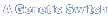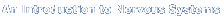Choose a Title A Genetic Switch Intro to Nervous Systems Epigenetics From a to alpha Genes and Signals Transcriptional RegulationClick on the highlighted text below to see the Chapter Figures

## Epigenetics

 Chapter 02 figures: Figure 01 (jpg) (PDF) Chapter 03 figures: Figure 02 (jpg) (PDF) Figure 03 (jpg) (PDF) Figure 04 (jpg) (PDF) Figure 05 (jpg) (PDF) Figure 06 (jpg) (PDF) Figure 07 (jpg) (PDF) Figure 08 (jpg) (PDF) Figure 09 (jpg) (PDF) Figure 10 (jpg) (PDF) Figure 11 (jpg) (PDF) Figure 12 (jpg) (PDF) Figure 13 (jpg) (PDF) Figure 14 (jpg) (PDF) Figure 15 (jpg) (PDF) Figure 16 (jpg) (PDF) Figure 17 (jpg) (PDF) Figure 18 (jpg) (PDF) Figure 19 (jpg) (PDF) Figure 20 (jpg) (PDF) Figure 21 (jpg) (PDF) Chapter 04 figures: Figure 01 (jpg) (PDF) Figure 02 (jpg) (PDF) Figure 03 (jpg) (PDF) Figure 04 (jpg) (PDF) Figure 05 (jpg) (PDF) Figure 06 (jpg) (PDF) Figure 07 (jpg) (PDF) Figure 08 (jpg) (PDF) Figure 09 (jpg) (PDF) Figure 10 (jpg) (PDF) Figure 11 (jpg) (PDF) Chapter 05 figures: Figure 01 (jpg) (PDF) Figure 02 (jpg) (PDF) Figure 03 (jpg) (PDF) Figure 04 (jpg) (PDF) Figure 05 (jpg) (PDF) Figure 06 (jpg) (PDF) Figure 07 (jpg) (PDF) Chapter 06 figures: Figure 01 (jpg) (PDF) Figure 02 (jpg) (PDF) Figure 03 (jpg) (PDF) Figure 04 (jpg) (PDF) Figure 05 (jpg) (PDF) Figure 06 (jpg) (PDF) Figure 07 (jpg) (PDF) Figure 08 (jpg) (PDF) Figure 09 (jpg) (PDF) Figure 10 (jpg) (PDF) Figure 11 (jpg) (PDF) Figure 12 (jpg) (PDF) Figure 13 (jpg) (PDF) Figure 14 (jpg) (PDF) Figure 15 (jpg) (PDF) Figure 16 (jpg) (PDF) Chapter 07 figures: Figure 01 (jpg) (PDF) Figure 02 (jpg) (PDF) Figure 03 (jpg) (PDF) Figure 04 (jpg) (PDF) Figure 05 (jpg) (PDF) Figure 06 (jpg) (PDF) Figure 07 (jpg) (PDF) Figure 08 (jpg) (PDF) Figure 09 (jpg) (PDF) Figure 10 (jpg) (PDF) Chapter 08 figures: Figure 01 (jpg) (PDF) Figure 02 (jpg) (PDF) Figure 03 (jpg) (PDF) Figure 04 (jpg) (PDF) Figure 05 (jpg) (PDF) Figure 06 (jpg) (PDF) Figure 07 (jpg) (PDF) Chapter 09 figures: Figure 01 (jpg) (PDF) Figure 03 (jpg) (PDF) Chapter 10 figures: Figure 01 (jpg) (PDF) Figure 02 (jpg) (PDF) Figure 03 (jpg) (PDF) Figure 04 (jpg) (PDF) Figure 05 (jpg) (PDF) Figure 06 (jpg) (PDF) Figure 07 (jpg) (PDF) Figure 08 (jpg) (PDF) Chapter 11 figures: Figure 01 (jpg) (PDF) Figure 02 (jpg) (PDF) Figure 03 (jpg) (PDF) Figure 04 (jpg) (PDF) Figure 05 (jpg) (PDF) Figure 06 (jpg) (PDF) Figure 07 (jpg) (PDF) Figure 08 (jpg) (PDF) Figure 09 (jpg) (PDF) Chapter 12 figures: Figure 01 (jpg) (PDF) Figure 02 (jpg) (PDF) Figure 03 (jpg) (PDF) Figure 04 (jpg) (PDF) Figure 05 (jpg) (PDF) Figure 06 (jpg) (PDF) Figure 07 (jpg) (PDF) Figure 08 (jpg) (PDF) Chapter 13 figures: Figure 01 (jpg) (PDF) Figure 02 (jpg) (PDF) Figure 03 (jpg) (PDF) Figure 04 (jpg) (PDF) Figure 05 (jpg) (PDF) Figure 06 (jpg) (PDF) Figure 07 (jpg) (PDF) Figure 08 (jpg) (PDF) Figure 09 (jpg) (PDF) Chapter 14 figures: Figure 01 (jpg) (PDF) Figure 02 (jpg) (PDF) Figure 03 (jpg) (PDF) Figure 04 (jpg) (PDF) Figure 05 (jpg) (PDF) Figure 06 (jpg) (PDF) Figure 07 (jpg) (PDF) Figure 08 (jpg) (PDF) Figure 09 (jpg) (PDF) Figure 10 (jpg) (PDF) Figure 11 (jpg) (PDF) Chapter 15 figures: Figure 01 (jpg) (PDF) Figure 02 (jpg) (PDF) Figure 03 (jpg) (PDF) Figure 04 (jpg) (PDF) Figure 05 (jpg) (PDF) Figure 06 (jpg) (PDF) Figure 07 (jpg) (PDF) Figure 08 (jpg) (PDF) Chapter 16 figures: Figure 01 (jpg) (PDF) Figure 02 (jpg) (PDF) Figure 03 (jpg) (PDF) Figure 04 (jpg) (PDF) Figure 05 (jpg) (PDF) Figure 07 (jpg) (PDF) Figure 08 (jpg) (PDF) Figure 09 (jpg) (PDF) Chapter 17 figures: Figure 01 (jpg) (PDF) Figure 02 (jpg) (PDF) Figure 03 (jpg) (PDF) Figure 04 (jpg) (PDF) Figure 05 (jpg) (PDF) Figure 06 (jpg) (PDF) Figure 07 (jpg) (PDF) Figure 08 (jpg) (PDF) Figure 09 (jpg) (PDF) Chapter 18 figures: Figure 01 (jpg) (PDF) Figure 02 (jpg) (PDF) Figure 03 (jpg) (PDF) Figure 04 (jpg) (PDF) Figure 05 (jpg) (PDF) Figure 06 (jpg) (PDF) Chapter 19 figures: Figure 01 (jpg) (PDF) Figure 02 (jpg) (PDF) Figure 03 (jpg) (PDF) Figure 04 (jpg) (PDF) Figure 05 (jpg) (PDF) Figure 06 (jpg) (PDF) Figure 07 (jpg) (PDF) Chapter 20 figures: Figure 01 (jpg) (PDF) Figure 02 (jpg) (PDF) Figure 03 (jpg) (PDF) Figure 04 (jpg) (PDF) Figure 05 (jpg) (PDF) Figure 06 (jpg) (PDF) Figure 07 (jpg) (PDF) Figure 08 (jpg) (PDF) Figure 09 (jpg) (PDF) Chapter 21 figures: Figure 01 (jpg) (PDF) Figure 02 (jpg) (PDF) Figure 03 (jpg) (PDF) Figure 04 (jpg) (PDF) Figure 05 (jpg) (PDF) Figure 06 (jpg) (PDF) Figure 07 (jpg) (PDF) Figure 08 (jpg) (PDF) Figure 09 (jpg) (PDF) Figure 10 (jpg) (PDF) Figure 11 (jpg) (PDF) Chapter 22 figures: Figure 01 (jpg) (PDF) Figure 02 (jpg) (PDF) Figure 03 (jpg) (PDF) Figure 04 (jpg) (PDF) Figure 05 (jpg) (PDF) Figure 06 (jpg) (PDF) Figure 07 (jpg) (PDF) Figure 08 (jpg) (PDF) Figure 09 (jpg) (PDF) Figure 10 (jpg) (PDF) Chapter 23 figures: Figure 01 (jpg) (PDF) Figure 02 (jpg) (PDF) Figure 03 (jpg) (PDF) Figure 04 (jpg) (PDF) Figure 05 (jpg) (PDF) Figure 06 (jpg) (PDF) Figure 07 (jpg) (PDF) Chapter 24 figures: Figure 01 (jpg) (PDF) Figure 02 (jpg) (PDF) Figure 03 (jpg) (PDF) Figure 04 (jpg) (PDF) Figure 05 (jpg) (PDF) Figure 06 (jpg) (PDF) Figure 07 (jpg) (PDF) Figure 08 (jpg) (PDF)

 © 2002 by Cold Spring Harbor Laboratory Press. All rights reserved.No part of these pages, either text or image, may be used for any purpose other than personal use. Therefore, reproduction, modification, storage in a retrieval system, or retransmission, in any form or by any means, electronic, mechanical, or otherwise, for reasons other than personal use, is strictly prohibited without prior written permission.